# De Morgan's Theorems

We use De Morgan’s theorems to solve the expressions of Boolean Algebra. It is a very powerful tool used in digital design. This theorem explains that the complements of the products of all the terms are equal to the sums of the complements of each and every term. Likewise, the complements of the sums of all the terms are equal to the products of the complements of each and every term.

## What are De Morgan’s Theorems?

Two of the theorems were suggested by De Morgan that are extremely useful for Boolean Algebra. These two theorems have been discussed below in this article:

### Theorem 1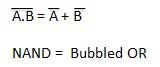• The LHS (left-hand side) of this theorem represents the NAND gate that has inputs A and B. On the other hand, the RHS (right-hand side) of this theorem represents the OR gate that has inverted inputs.
• The OR gate here is known as a Bubbled OR.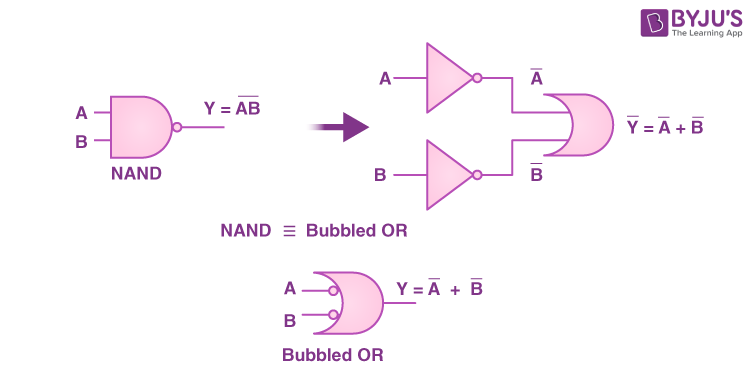Here is a table that shows the verification of the first theorem of De Morgan: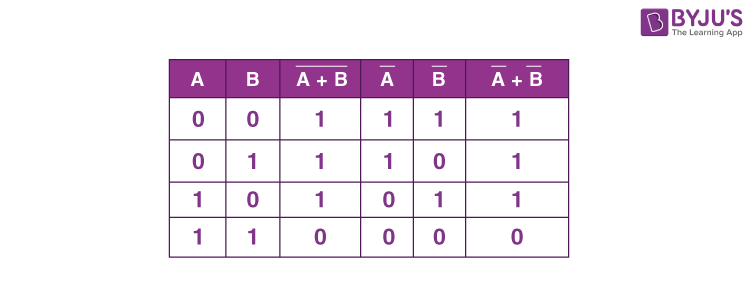### Theorem 2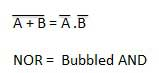• The left-hand side of this theorem represents the NOR gate that has inputs A and B. On the other hand, the right-hand side represents the AND gate that has inverted inputs.
• The AND gate here is known as a Bubbled AND.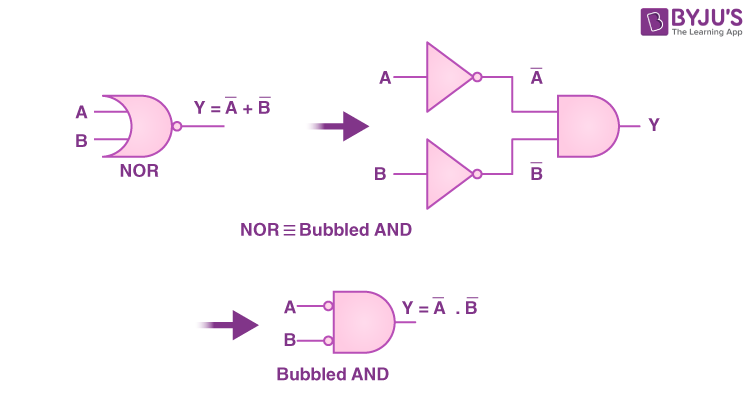Here is a table that shows the verification of the second theorem of De Morgan: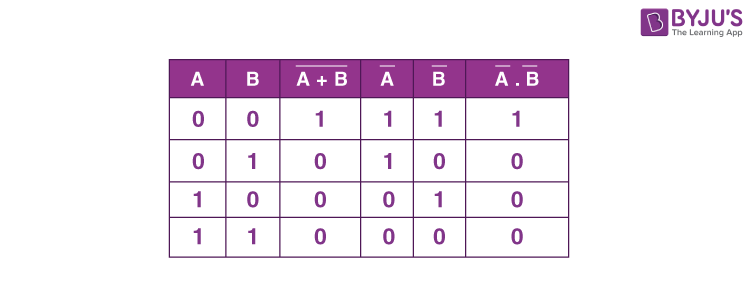## Practice Problems on De Morgan’s Theorems

1. Which of these is De-Morgan’s law?

a) A ∧ (B v C) Ξ (A ∧ B) v (A ∧ C)

b) ~(A ∧ C) Ξ ~A v ~C, ~(A v C) Ξ ~A ∧ ~C

c) A v ~A Ξ True, A ∧ ~A Ξ False

d) None of the above

Answer – (b) ~(A ∧ C) Ξ ~A v ~C, ~(A v C) Ξ ~A ∧ ~C

2. What would be the dual of (W ∧ X) v (Y ∧ Z)?

a) (W V X) v (Y v Z)

b) (W V X) ^ (Y v Z)

c) (W V X) v (Y ∧ Z)

d) (W ∧ X) v (Y v Z)

Answer – (b) (W V X) ^ (Y v Z)

3. What would be the negation of statement (M ∧ N) → (N ∧ O)

a) (M ∧ N) →(~N ∧ ~O)

b) ~(M ∧ N) v ( N v O)

c) ~(M → N) →(~N ∧ O)

d) None of the above

Answer – (a) (M ∧ N) →(~N ∧ ~O)

## FAQs

Q1

### What is De Morgan known for?

De Morgan was one of the Cambridge mathematicians. He recognized that the nature of algebra is purely symbolic. He was very much aware of the possibility that some algebras differ from ordinary algebra.

Q2

### What are the implications of De Morgan’s theorem?

De Morgan’s theorems can be used when we want to prove that the NAND gate is equal to the OR gate that has inverted inputs and the NOR gate is equal to the AND gate that has inverted inputs. To reduce the expressions that have large bars, we must first break up these bars.

Q3

### What are the basic properties of Boolean algebra?

The three basic properties of Boolean algebra are commutative, associative, and distributive.

Q4

### What are De Morgan’s theorems?

We use De Morgan’s theorems to solve the expressions of Boolean Algebra. It is a very powerful tool used in digital design. This theorem explains that the complements of the products of all the terms are equal to the sums of the complements of each and every term. Likewise, the complements of the sums of all the terms are equal to the products of the complements of each and every term.

Keep learning and stay tuned to get the latest updates on GATE Exam along with GATE Eligibility CriteriaGATE 2023GATE Admit CardGATE SyllabusGATE Previous Year Question Paper, and more.

Also Explore,